# 基于PLC的非线性水箱的预测控制研究Research on Predictive Control of Nonlinear Water Tank Based on PLC

• 全文下载: PDF(963KB)    PP.310-317   DOI: 10.12677/DSC.2018.74035
• 下载量: 339  浏览量: 690

The industrial production process is often a large inertia, large delay, and nonlinear control object. It is difficult to achieve good control effect by conventional PID control. The prediction control can effectively solve the problems of nonlinearity, large delay and large inertia. This paper selects the typical industrial process object, namely the nonlinear water tank for predictive control research. Before the tank test, it is necessary to identify the object model and pre-define the necessary pa-rameters on MATLAB. The experimental results show that the constrained predictive control al-gorithm is superior to the traditional PID control in terms of control quality and adjustment time, and the robust performance of the algorithm is good.

1. 引言

2. 动态矩阵控制(DMC)

2.1. 基本原理介绍

DMC算法包含三个部分：预测模型、滚动优化、反馈校正  。其算法流程图如图1所示。

2.2. 基于黄金分割法的有约束预测控制器设计

2.2.1. 黄金分割算法介绍

(1) 确定收敛精度 $\epsilon$

(2) 从 $\left[a,b\right]$ 区间内选择 ${r}_{1},{r}_{2}\left({r}_{1}<{r}_{2}\right)$ 两点，然后将 $\left[a,b\right]$ 分成三段；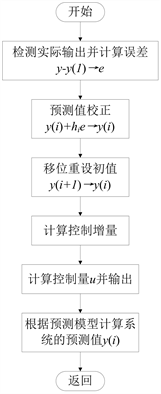Figure 1. Flow chart of unconstrained dynamic matrix control algorithm

(3) 判断 $f\left({r}_{1}\right)$$f\left({r}_{2}\right)$ 的大小，若 $f\left({r}_{1}\right)>f\left({r}_{2}\right)$ ，则收敛区间则需要更新：

$a={r}_{1}$

${r}_{1}={r}_{2}$

${r}_{2}=a+0.618×\left(b-a\right)$

$f\left({r}_{1}\right)>f\left({r}_{2}\right)$ ，则收敛区间的更新公式为：

$b={r}_{2}$

${r}_{2}={r}_{1}$

${r}_{1}=a+0.382×\left(b-a\right)$

(4) 若 $\frac{b-a}{a}<\epsilon$ 则停止迭代，极值点为 $\frac{a+b}{2}$ ，函数的极值为 $f\left(\frac{a+b}{2}\right)$ ；否则，返回步骤(3)继续迭代。

2.2.2. 基于黄金分割法的有约束预测控制器设计

$\mathrm{min}J\left(k\right)={‖{w}_{p}\left(k\right)-{\stackrel{˜}{y}}_{p0}\left(k\right)-{A}_{2}\Delta u\left(k\right)‖}_{Q}^{2}+{H}^{T}RH\Delta {u}^{2}\left( k \right)$

$\begin{array}{l}\Delta u\left(k\right)=\mathrm{arg}\mathrm{min}J\left(\Delta u\left(k\right)\right)\\ s.t.\Delta u\left(k+i\right)\in \left[\Delta {u}_{\mathrm{min}},\Delta {u}_{\mathrm{max}}\right],\Delta {u}_{\mathrm{min}}<0,\Delta {u}_{\mathrm{max}}>0\\ u\left(k+i\right)\in \left[{u}_{\mathrm{min}},{u}_{\mathrm{max}}\right],{u}_{\mathrm{min}}\ge 0,\Delta {u}_{\mathrm{max}}>0\\ \Delta u\left(k+i\right)={\gamma }^{i}\Delta u\left(k\right),1\le i\le M-1\end{array}$

$\begin{array}{l}u\left(k\right)=\Delta u\left(k\right)+u\left(k-1\right)\\ u\left(k+1\right)=\gamma \Delta u\left(k\right)+\Delta u\left(k\right)+u\left(k-1\right)\\ u\left(k+M-1\right)={R}_{i}\Delta u\left(k\right)+u\left(k-1\right)\end{array}$

$\Delta u\left(k\right)\in \left\{\begin{array}{c}\left[\mathrm{max}\left(\Delta {u}_{\mathrm{min}},\frac{{u}_{\mathrm{min}}-u\left(k-1\right)}{{R}_{M-1}}\right),\mathrm{min}x\left(\Delta {u}_{\mathrm{max}},\frac{{u}_{\mathrm{max}}-u\left(k-1\right)}{{R}_{M-1}}\right)\right],\gamma <1\\ \left[\mathrm{max}\left(\frac{\Delta {u}_{\mathrm{min}}}{{\gamma }^{M-1}},\frac{{u}_{\mathrm{min}}-u\left(k-1\right)}{{R}_{M-1}}\right),\mathrm{min}x\left(\frac{\Delta {u}_{\mathrm{max}}}{{\gamma }^{M-1}},\frac{{u}_{\mathrm{max}}-u\left(k-1\right)}{{R}_{M-1}}\right)\right],\gamma \ge 1\end{array}\right\}$

3. MATLAB仿真实例

3.1. 控制对象的辨识及参数的获取Figure 2. Flow chart of constrained predictive control algorithm based on golden section method

${G}_{\left(s\right)}=\frac{3.7766}{{\left(52.676s+1\right)}^{2}}$

3.2. DMC算法和PID算法的比较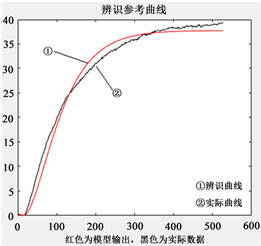Figure 3. Flying curve of nonlinear water tank under valve disturbance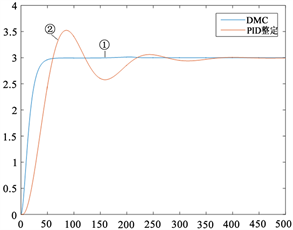Figure 4. Simulation results

4. 基于PLC的水箱控制试验

4.1. 实验设备介绍

A3030型艾默生过程自动化实训系统通过实际流体(自来水)的流量控制和液位控制来模拟对实际对象的控制和设备管理，它充分展示了DCS分散控制功能，体现了控制系统和现场仪表间的协同运作能力以及在PID回路之外建立设备管理平台为用户带来预诊断功能的先进理念，由于上水箱是一个圆筒状模型，一个具有典型非线性特征的对象，可以模拟很多实际工业过程被控对象。

4.2. 实验流程及实例

OVATION是美国开发的新型分散控制系统，它既包含过程控制技术，又吸收了企业管理信息技术，系统在硬件上采用了速度快、容量大的设备，保证系统的高性能运行；通讯网络使用标准的以太网，可靠性高、速度快、开放性高，提升其多任务、多数据的工作能力。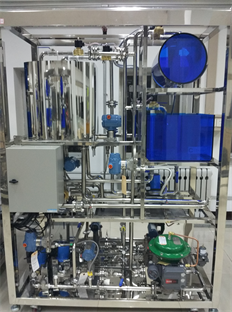Figure 5. A3030 process system physics experiment platformFigure 6. Experimental flow diagram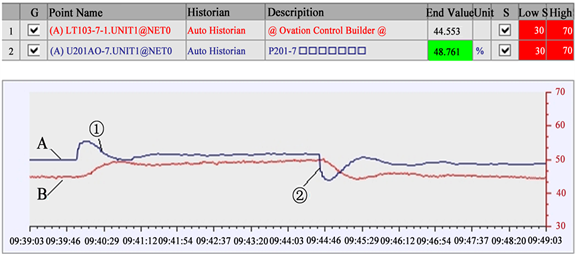Figure 7. Water tank level control trend

5. 结论

  石宇静. 复杂工业过程模型预测控制的研究[D]. 沈阳: 东北大学, 2009.  徐祖华. 模型预测控制理论及应用研究[D]. 杭州: 浙江大学, 2004.  席裕庚. 预测控制[M]. 第二版. 国防工业出版社, 2013: 2-3.  席裕庚, 李德伟, 林姝. 模型预测控制——现状与挑战[J]. 自动化学报, 2013(3): 222-236.  鲍荣, 何德峰, 郑凯华. 基于黄金分割法的阶梯式约束预测控制[J]. 上海交通大学学报, 2012, 46(12): 1940-1944.  陈薇, 吴刚, 阎镜予, 凌青, 郑涛. 阶梯式有约束非线性预测控制及其应用[J]. 系统仿真学报, 2007(18): 4226-4228+4234.  朱娜娜. 模型预测控制简化算法的研究[D]. 杭州: 浙江大学, 2011.  刘有飞, 吴刚, 魏衡华, 孙德敏. 阶梯式动态矩阵控制及在温度控制系统中的应用[J]. 中国科学技术大学学报, 2002(3): 92-97.